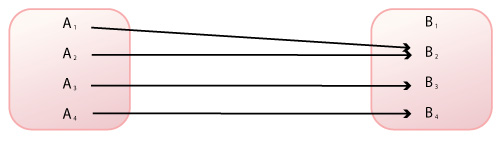Skip to content

Chapter 08 - further questions

For instructors:

More resources coming soon

Further questions for chapter 08

Q. Label the domain and range in the figure below (from Figure 8.4).Q. The above figure illustrates a mapping function. How many items in the range of a mapping function can be attached to each item in its domain?

Q. Explain the phrase "neurons that fire together, wire together."

Q. Identify some differences between artificial and real, biological neural networks.

Q. Explain why networks with hidden layers solve problems created by non-linearly separable functions.

Q. Explain the process of learning for a neural network, and do so by using the perceptron convergence rule.

Not already registered? Create an account now. ×